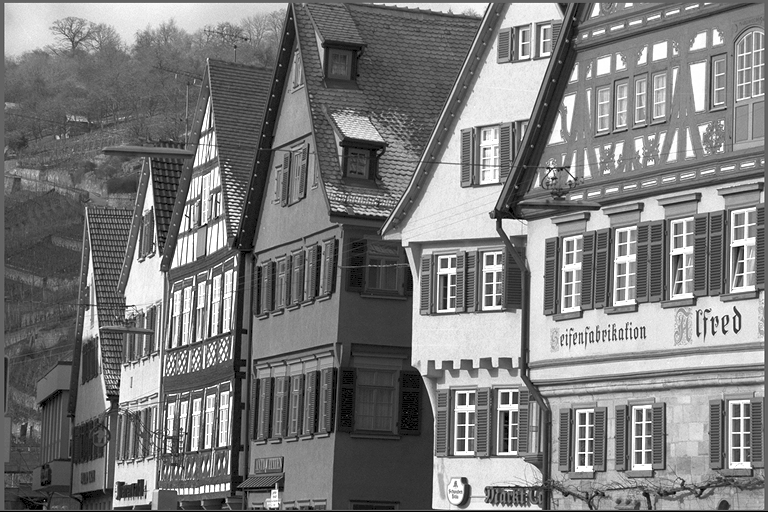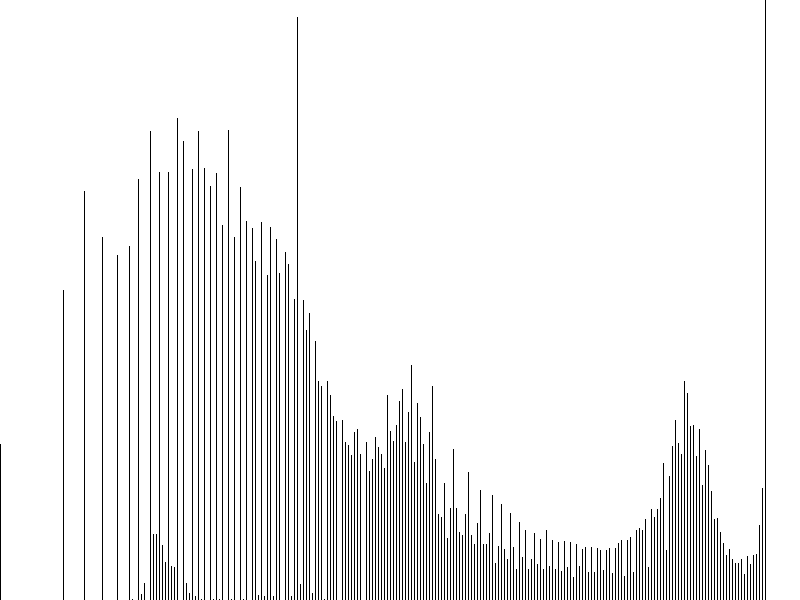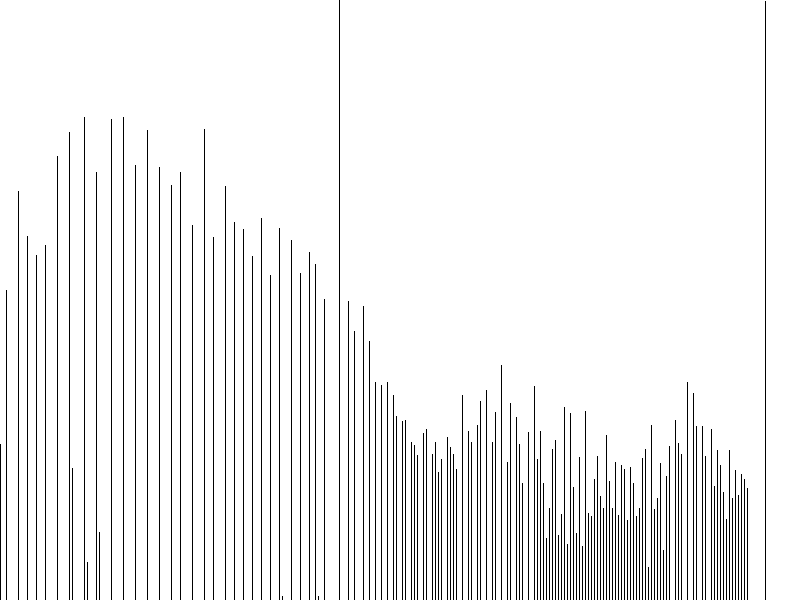## VPI - Vision Programming Interface

#### 2.3 Release

Equalize Histogram

# Overview

Histogram equalization is a method in image processing of contrast adjustment using the image's histogram. For more information, see .

Input Output# Implementation

This algorithm is trying to find a new mapping palette based on the histogram. Consider a grayscale image X, whose total number of gray levels in the image is L, Denote the histogram of the image as $$H_x$$. The probability of an occurrence of a pixel of level $$i$$ in the image is:

$p[i] = H_x[i] / N$

where:

• $$N$$ is total number of pixels in the image.

The cumulative distribution function corresponding to $$p[i]$$ is:

$cdf_x[i] = \sum_{j=0}^{i}p[j]$

Using the cumulative distribution function to transform the original pixel value to a new pixel value, the corresponding pixel value is:

$y[i] = \sum_{j=0}^{i}p[j] * L$

Example of image histogram after equalization:

Input Equalized Output# C API functions

For list of limitations, constraints and backends that implements the algorithm, consult reference documentation of the following functions:

Function Description
vpiSubmitEqualizeHist Equalize the histogram of the image.

# Usage

Language:
1. Import VPI module
import vpi
2. Use the CUDA backend to equalize the input image histogram, creating output VPI image with the result.
with vpi.Backend.CUDA:
output = input.eqhist()
1. Initialization phase
1. Include the header that defines the image equalize histogram function.
Declares functions that equalize the histogram of the source image.
2. Define the input image.
VPIImage input = /*...*/;
struct VPIImageImpl * VPIImage
A handle to an image.
Definition: Types.h:256
3. Create the output image.
int w, h;
vpiImageGetSize(input, &w, &h);
VPIImage output;
vpiImageCreate(w, h, VPI_IMAGE_FORMAT_U8, 0, &output);
#define VPI_IMAGE_FORMAT_U8
Single plane with one 8-bit unsigned integer channel.
Definition: ImageFormat.h:100
VPIStatus vpiImageCreate(int32_t width, int32_t height, VPIImageFormat fmt, uint64_t flags, VPIImage *img)
Create an empty image instance with the specified flags.
VPIStatus vpiImageGetSize(VPIImage img, int32_t *width, int32_t *height)
Get the image dimensions in pixels.
4. Since this algorithm needs temporary memory buffers, create the payload for it on the CUDA backend.
A handle to an algorithm payload.
Definition: Types.h:268
@ VPI_BACKEND_CUDA
CUDA backend.
Definition: Types.h:93
5. Create the stream where the algorithm will be submitted for execution.
VPIStream stream;
vpiStreamCreate(0, &stream);
struct VPIStreamImpl * VPIStream
A handle to a stream.
Definition: Types.h:250
VPIStatus vpiStreamCreate(uint64_t flags, VPIStream *stream)
Create a stream instance.
2. Processing phase
1. Submit the algorithm to the stream using the CUDA backend, along with all parameters.
Equalize the histogram of the image.
2. Optionally, wait until the processing is done.
vpiStreamSync(stream);
VPIStatus vpiStreamSync(VPIStream stream)
Blocks the calling thread until all submitted commands in this stream queue are done (queue is empty)...
3. Cleanup phase
1. Free resources held by the stream and the input and output images.
vpiImageDestroy(output);
void vpiImageDestroy(VPIImage img)
Destroy an image instance.
Deallocates the payload object and all associated resources.
void vpiStreamDestroy(VPIStream stream)
Destroy a stream instance and deallocate all HW resources.

For more information, see Equalize Image Histogram in the "C API Reference" section of VPI - Vision Programming Interface.

# Performance

For information on how to use the performance table below, see Algorithm Performance Tables.
Before comparing measurements, consult Comparing Algorithm Elapsed Times.
For further information on how performance was benchmarked, see Performance Benchmark.

-